Math

• Home
• Programmes
• Math

JEI Math develops interest and confidence in math through
mathematical thinking, creativity and problem-solving skills by learning
based on concept and principle understanding.

JEI Math offers a complete programme for kindergarden to year 9 and
each level of the JEI Math programme is designed with specific learning
objectives and provides a step-by-step
approach which makes learning easy for everyone.

The JEI Math curriculum is aligned with the State
Standards, covering all major domains:
Number Concepts, Operations, Geometry,
Measurement, Data Analysis, and more.### Key Features of JEI Math

• 1

Encourage conceptual
understanding through
easy and small steps.

• 2

Build a strong understanding of
mathematical
thinking upon all
domains.

• 3

Strive to build
Self-Learning behaviour along
with the programmed workbooks
and diagnostic system.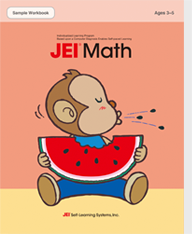View Sample

This is a sample workbook
that shows various
parts of the actual Math
workbooks used at JEI.Learning Objective Chart
This is a chart that shows the learning objectives for both Math and Problem Solving Math programmes.
VIEW MORE

### Math Level

Level A

• Trace and draw shapes
• Recognise and write numbers 1-5
• Count numbers 1-10
• Compare length, height, size, speed, and quantity
• Compose and decompose numbers 2-5
• Learn the concept of order
•••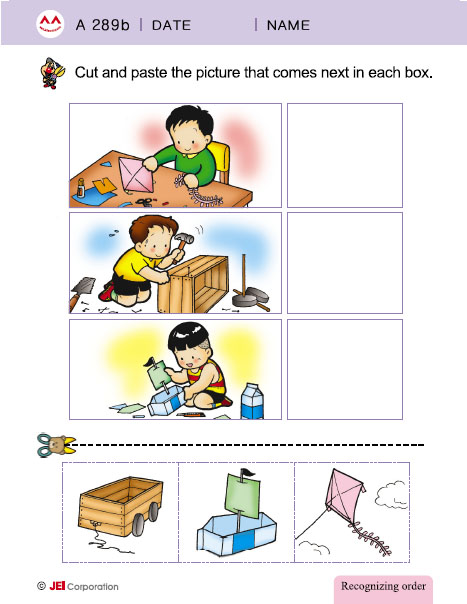•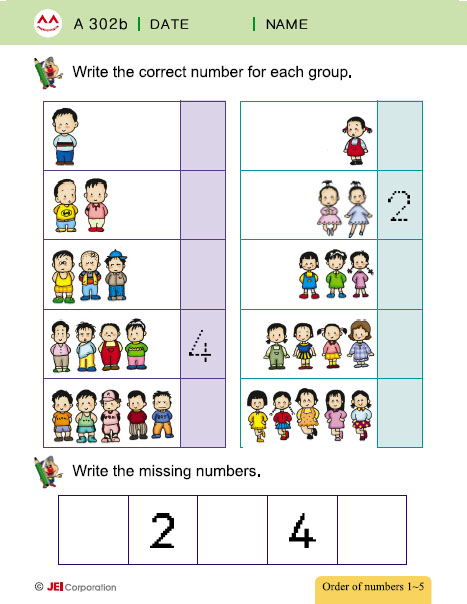•Level B

• Learn to recognise different figures
• Compare and classify different objects
• Recognise and write numbers 1-9
• Tell the order of numbers 1-9
• Compose & decompose numbers to 6
• Introduce simple addition and subtraction concepts
••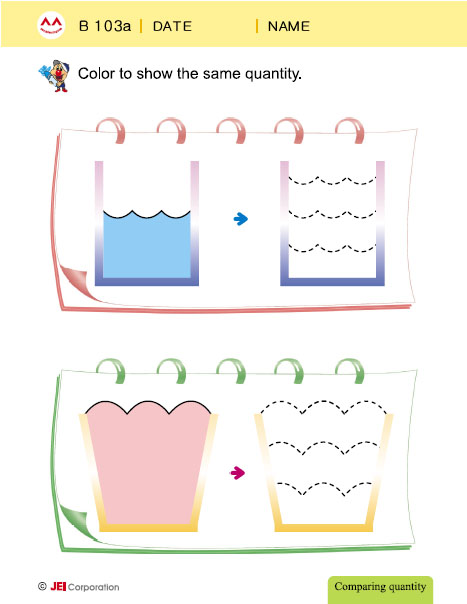•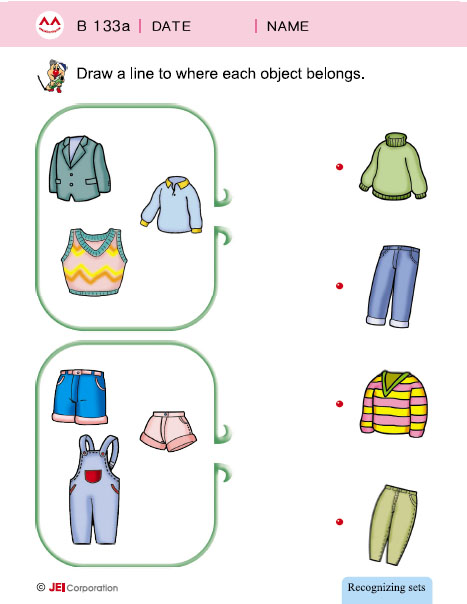•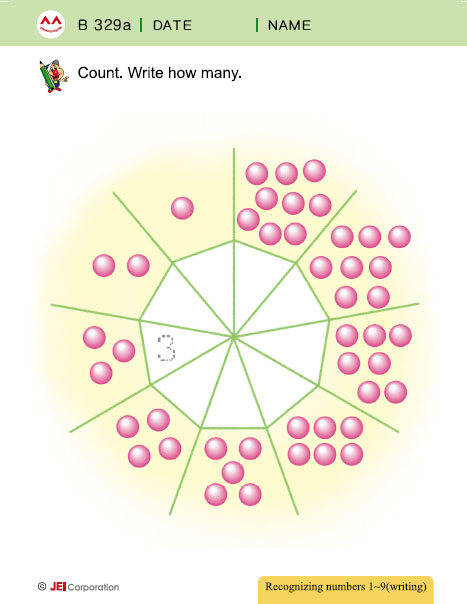•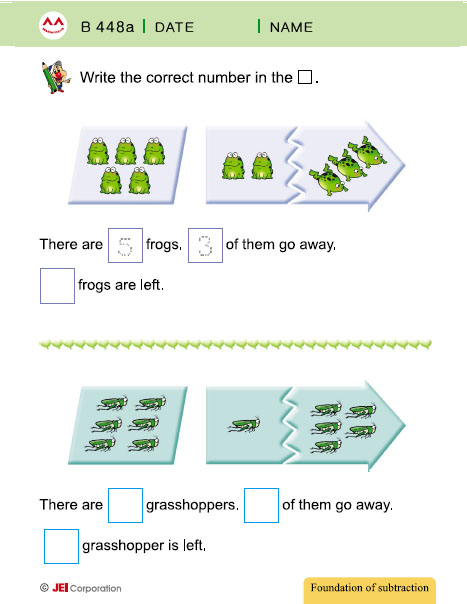Level C

• Group shapes by size and colour
• Learn expressions to compare quantities and sizes
• Learn to recognise and write numbers 1-20
• Learn to recognise ordinal numbers
• Compose and decompose numbers to 10
• Addition and subtraction to 5
• Learn to recognise positions of an object
••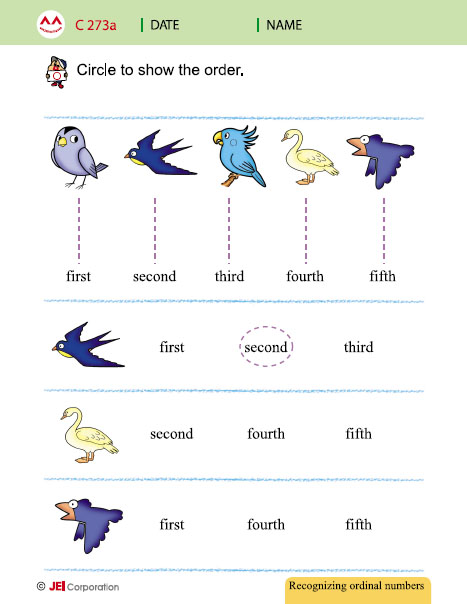•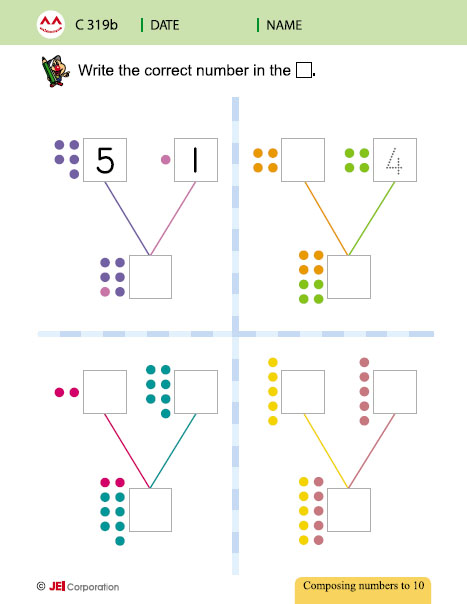••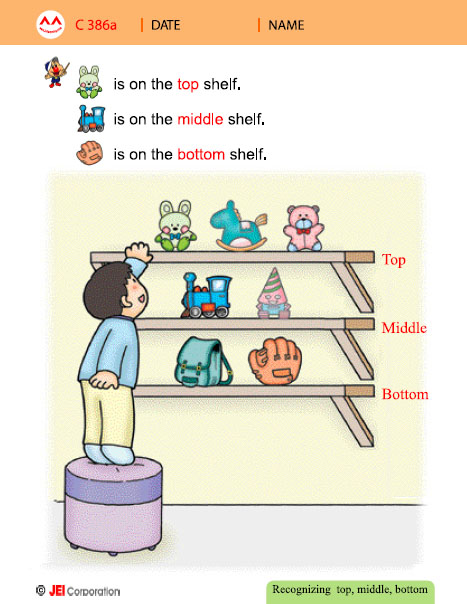Level D

• Number operation of addition and subtraction
• Learn to recognise & compare numbers 0-99
• Comprehend place values of ones and tens digits
• Learn to recognise 3-D and 2-D shapes
• Learn to tell time and read a calendar
• Learn to recognise coins and their value
••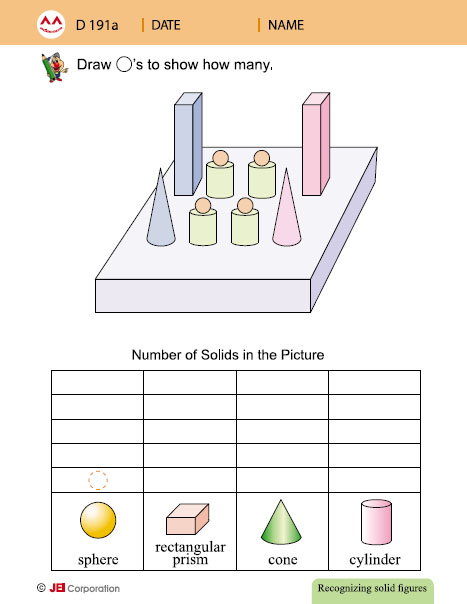•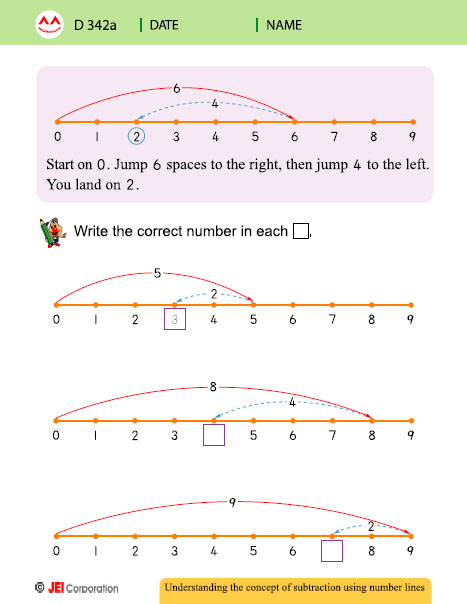•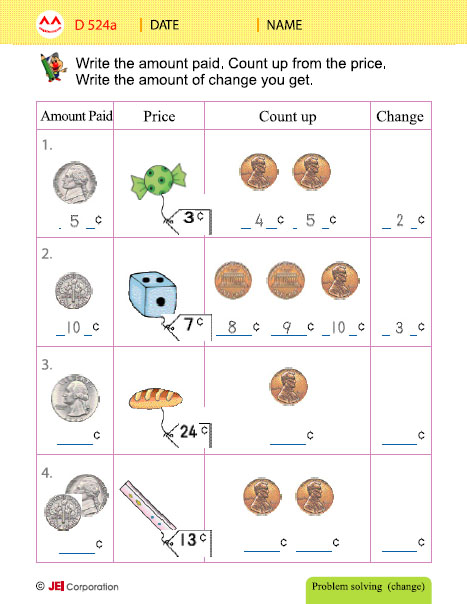•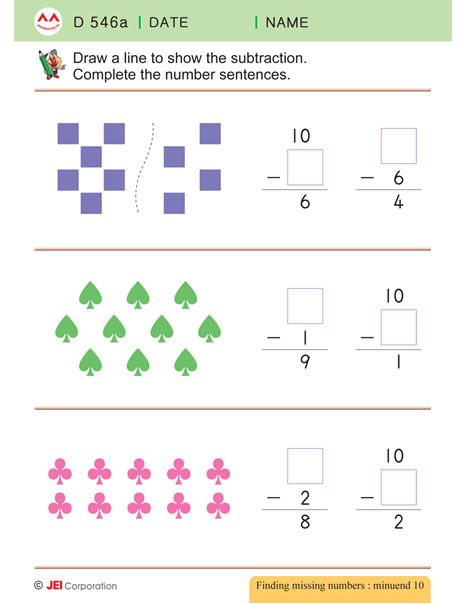Level E

• Learn place values of 3 digit numbers
• Introduce estimation and rounding concepts
• Introduce addition and subtraction of 2 digit numbers with regrouping
• Understand the terms of geometry (line, vertex, etc.)
• Tell time to each 5 minutes and read calendar
• Introduce addition and subtraction of units of length
•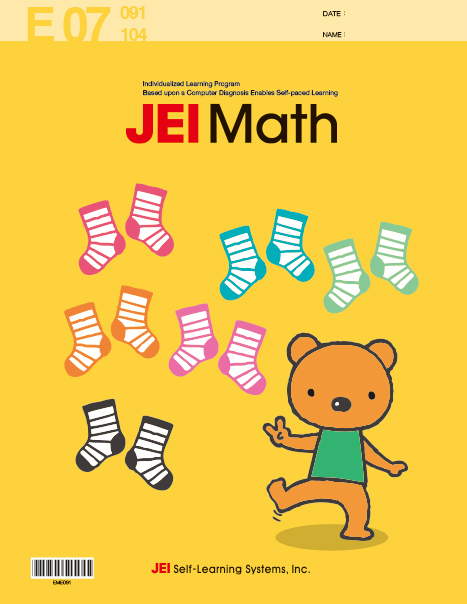••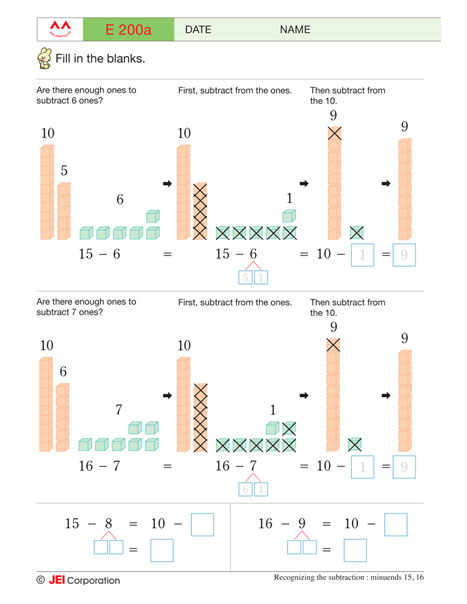•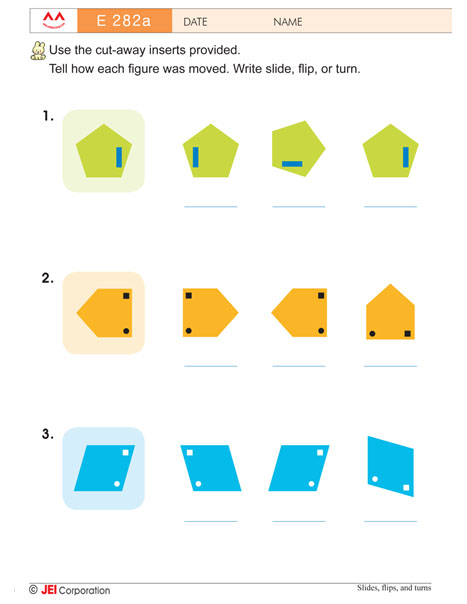•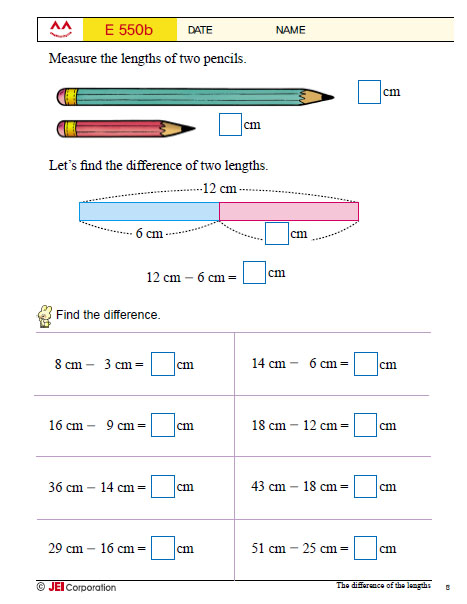Level F

• Introduce multiplication and division of 1 digit numbers
• Learn place values of 6 digit numbers
• Addition and subtraction of 3 to 6 digits with regrouping
• Tell time to each 1 minute
• Introduce the concepts of different units of length
• Learn to read different types of graphs
• Introduce the concept of probabilities
•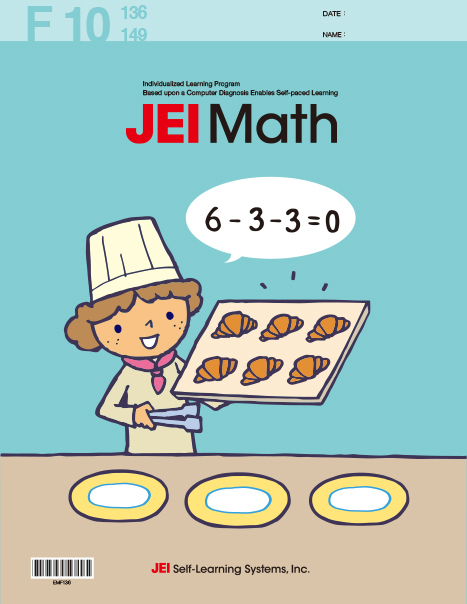••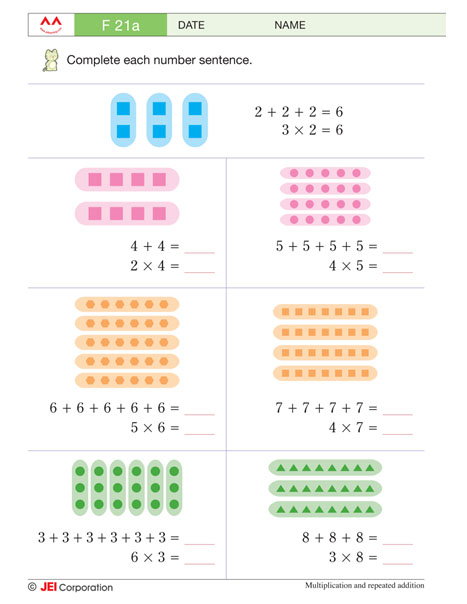•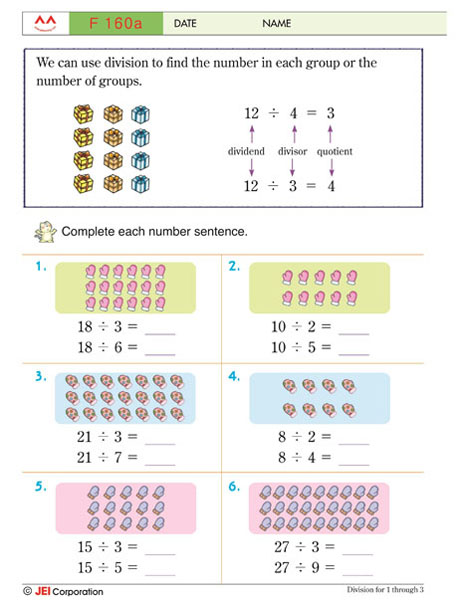•Level G

• Introduce fractions and decimals
• Understand multiplication of 3 digit numbers by 2 digit numbers
• Understand division with up to 5 digit dividends and 1 digit divisors
• Identify different types of triangles by given angles
• Introduce the measurement of the perimeter of a figure
• Recognise units of capacities and weights
• Addition and subtraction of like fractions and decimals
•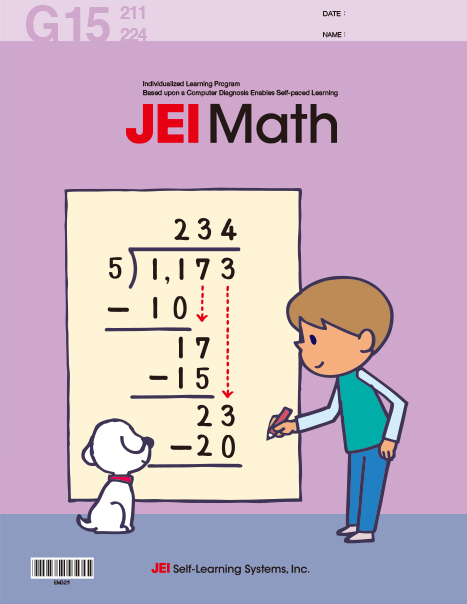••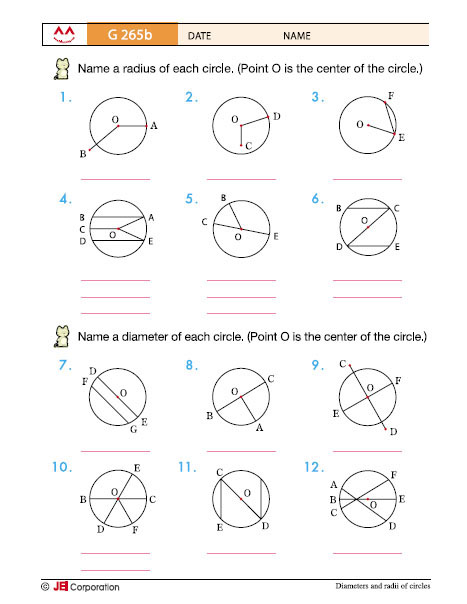•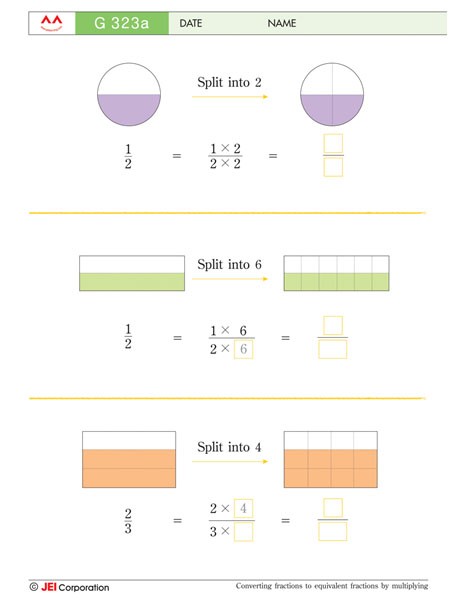•Level H

• Learn place values of large numbers
• Introduce degree of angles along with perpendicular and parallel lines
• Learn properties of triangles and quadrilaterals
• Division of 2 to 5 digit numbers by 2 digit numbers
• Addition and subtraction of unlike fractions
• Introduce multiplication and division of fractions
• Addition and subtraction of times up to seconds
• Finding the areas of plane figures
•••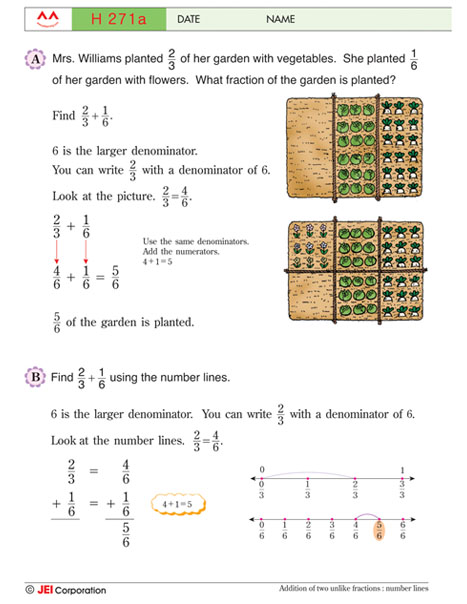••Level I

• Differentiate between prime and composite numbers
• Use prime factorisation to find GCF and LCM
• Introduce different methods to reduce fractions
• Division of decimals
• Understand the relationship among capacity, volume, and weight
• Learn mixed calculation of four operations with fractions and decimals
• Introduce ratios and percentages
••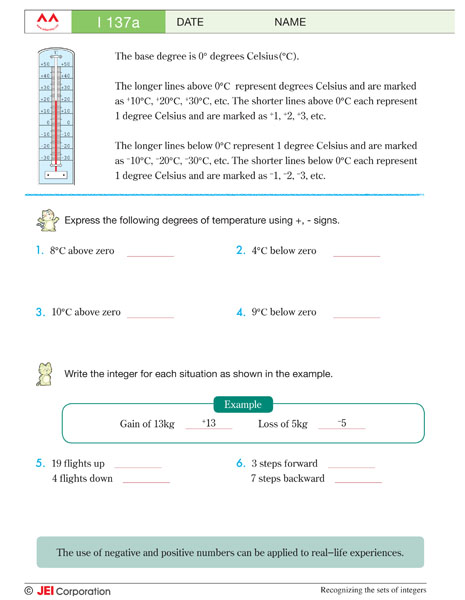•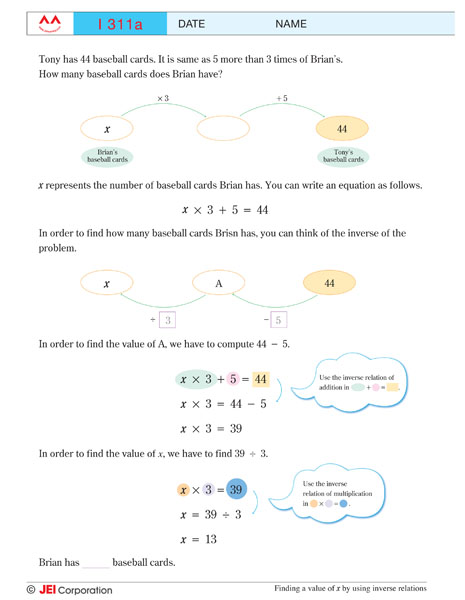••Level J

• Learn the concept of absolute values
• Introduce the concept of power, exponent, and base
• Learn about factors and multiples
• Translate phrases and sentences into mathematical expressions, equations, and inequalities
• Solve two-step and multi-step equations and inequalities
• Develop a conceptual understanding of area of a circle and volume of spheres
• Create word problems with given rates
• Learn to graph linear equations and inequalities
•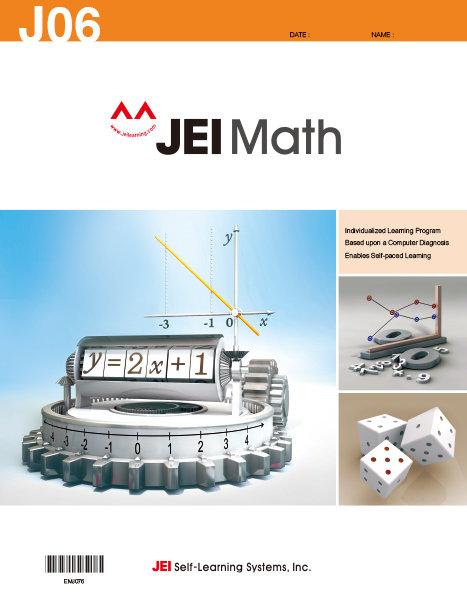•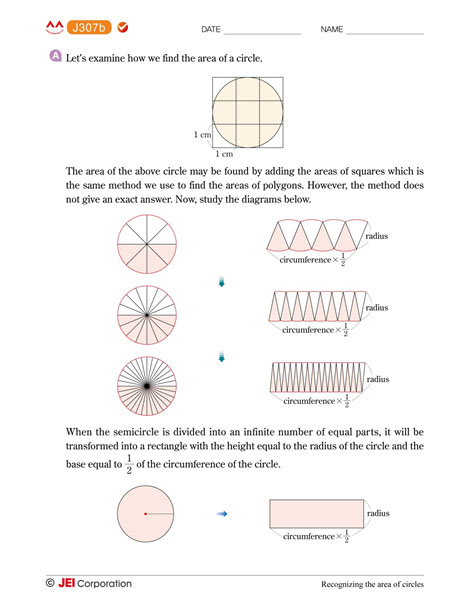•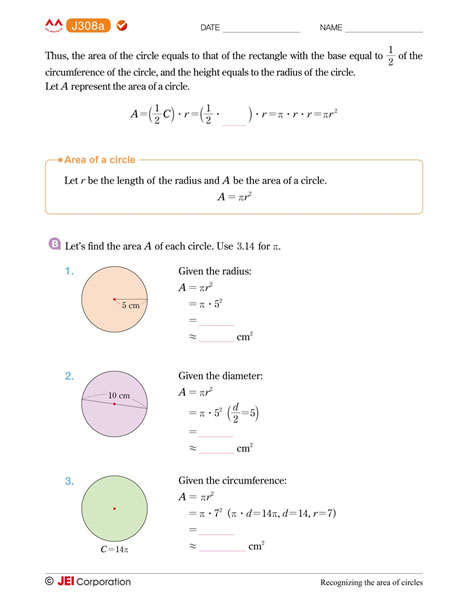•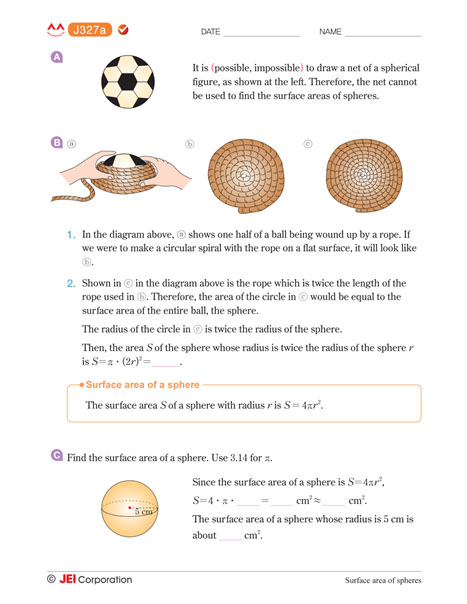•Level K

• Develop a conceptual understanding of slope by using multiple representations
• Solve systems of linear equations by graphing, elimination, and substitution methods
• Learn to multiply polynomials
• Learn to factor polynomials
• Solve a real world problem involving quadratic equation
• Understand the characteristics of rational functions by graphing
• Learn the property of probability
•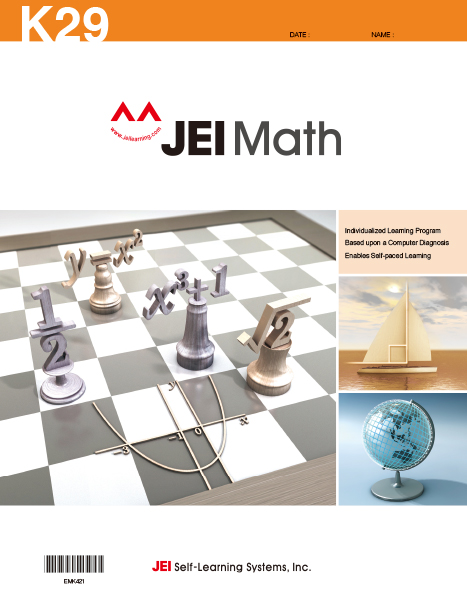•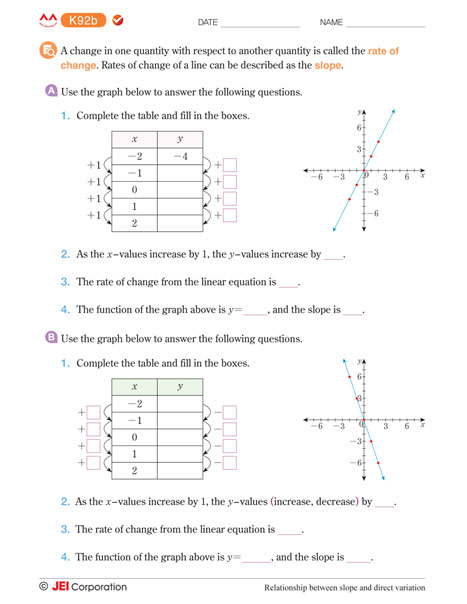••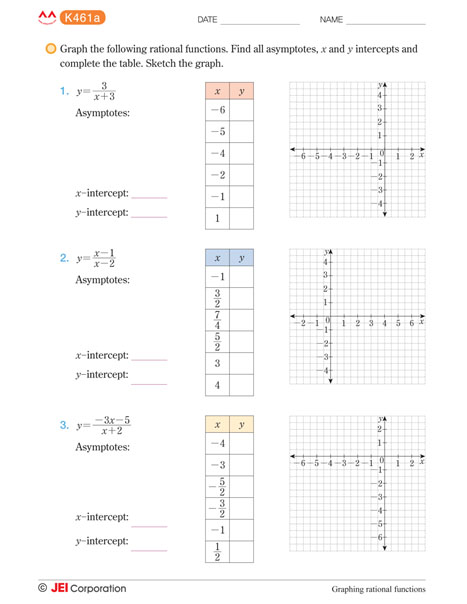•Level L

• Understand the different types of quadrilaterals
• Introduce frustum of pyramids
• Learn three different types of transformations: translation, reflection, and rotation
• Proving two congruent triangles in two-column proof using congruence postulates and theorems
• Learn the relations between the trigonometric ratios to solve for unknown sides or angles of triangles
• Identifying the relationships between a line and a plane
•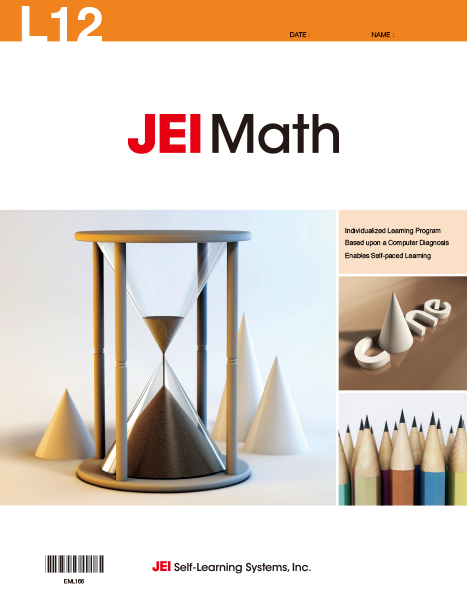•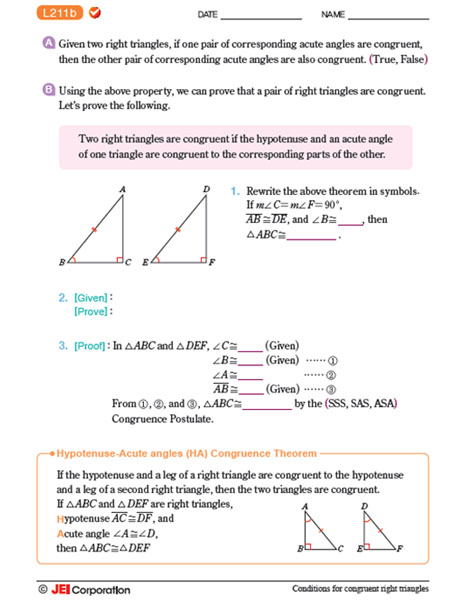•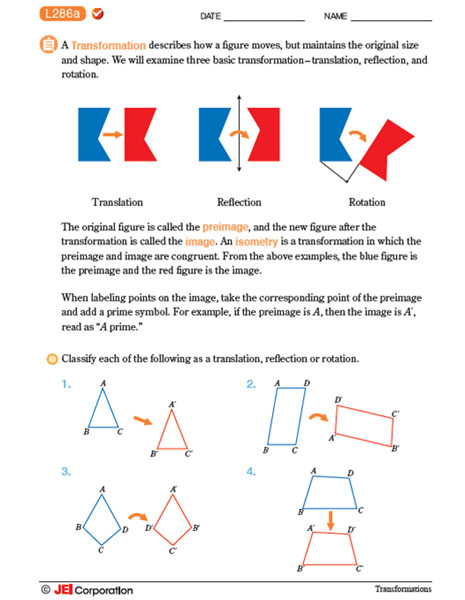•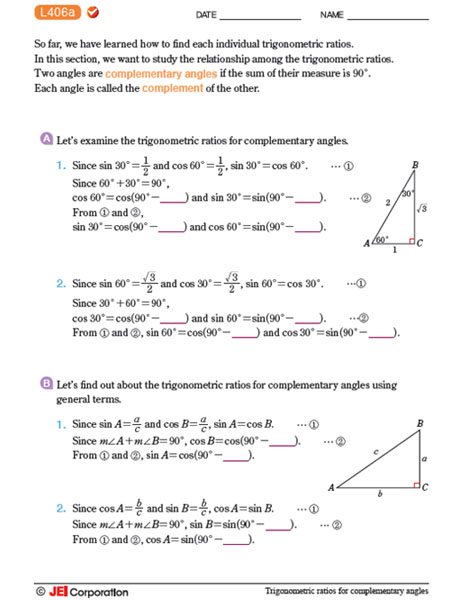•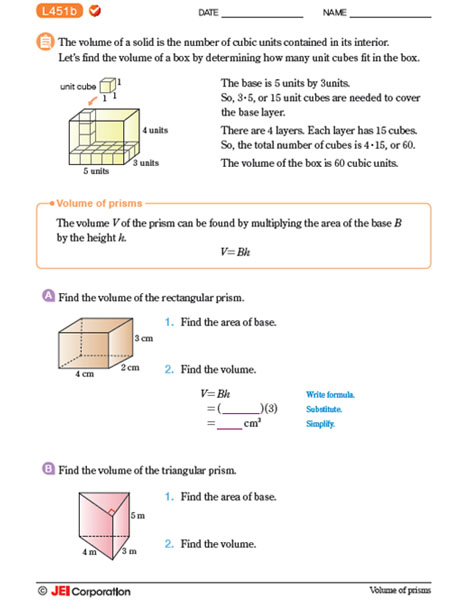# JEI Learning Centre International

JEI is a leader in global education and has been helping children for over 40 years in achieving academic success.

USA
JEI North American
4465 Wilshire Blvd., Suite 302,
Los Angeles, CA 90010
JEI NJ Corporate Office
440 Sylvan Ave., Suite 212,
Englewood Cliffs, NJ 07632
JEI NJ Corporate Office
440 Sylvan Ave., Suite 212,
Englewood Cliffs, NJ 07632
Hong Kong
JEI Hong Kong, LTD.
401-402, 4th Floor, Cheuk Nang Centre, No 9 Hillwood Road, Tsim Sha Tsui
India
JEI Learning Centres
8A Mohini Mohan Road Unit 1A, Siddharth Arcade Kolkata, West Bengal 700020
Australia
JEI Self-Learning Systems Pty LTD
Shop 42, Lemon Grove Shopping Centre, 427-441 Victoria Avenue, Chatswood, NSW 2067
New Zealand
JEI Self-Learning Systems Limited
Building A, 1st Floor, 20 Link Drive, Wairau Valley, Auckland 0627
Saudi Arabia
JEI Learning Centre
Mohammadyiyah Dist. Near Continetal School, 21473 Jeddah